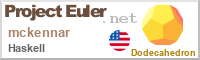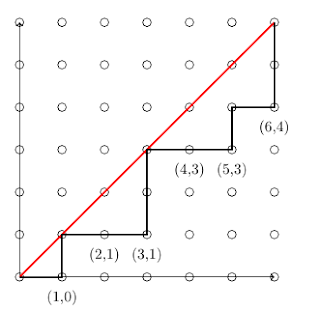## Posts

Showing posts from December, 2014

### Top Ten Reasons I Like Haskell

A few weeks ago I wrote about some of the differences between functional languages (like Haskell) and imperative languages (like C or Java). While I made some good points in this post, I don't think I gave enough credit to Haskell. After learning more about Haskell, it has become my favorite programming language. In this blog post, I will talk about some of reasons I like Haskell. Please keep in mind that I am relatively new to Haskell and the contents of this blog post are about my experience as a beginner. That being said, the intended audience of this blog post is people coming from an OOP background that are looking for something better. Purity In a Purely Functional language like Haskell, it's impossible to mutate objects in memory. While this may seem like a limitation, I assure you it is not. In Haskell, functions must take input and return output, but they may not have side effects. This has great benefits as it makes it easier to reason about code, and the compiler

### Multi-Core Programming with Java

Processors speeds are no longer growing at the rate we've grown accustomed to. Moore's law states that computers double in speed every 18 months. Moore's law is still alive and well, but we just need to find alternate ways to speed up computers. That's where multi-core programming comes into play. The idea behind it is that 2 heads are better than one and in the right situation 2 processors can work on the same problem to solve it twice as fast. Parallel programming is becoming increasingly important these days and there are many different flavors of it including distributed computing, shared memory computing, and GPU computing. Today I am going to focus on shared memory computing, or using multi-core computers rather than many single core computers. This blog post is intended to serve as a beginners guide to parallel programming with Java. It is recommended that the reader have some java experience and is familiar with some of the features of Java 8 (namely lambda expr

### Mathematics and Darts!

In this blog post I am going to talk about one of my favorite math problems. When I first solved this problem, I developed a method that can be generalized to a lot of other similar problems. The problem is as follows: Alice and Bob take turns throwing darts at a dartboard (with Alice going first). At each turn, Alice will try to get it closer to the center than Bob's last throw and vice versa. The game ends when one of the players doesn't get it closer than the other player's previous throw. Alice and Bob are equally skilled at throwing darts and they are guaranteed to hit the dart board. What is the probability that Alice wins? To help you visualize this problem, I created a simple program in Racket (then compiled it to JavaScript using whalesong .) You can visualize the game being played by pressing the Right Arrow Key (make sure the frame is in focus). You can speed up the simulation by pressing the Space Bar (you can pause the simulation by pressing it again.)

### 200th Problem Solved!Today I solved my 200th Project Euler problem! Project Euler is a great website that posts weekly programming challenges. It is a great way to learn a new programming language, master the art of algorithm design, and learn about fascinating math concepts. In light of this milestone, I decided to do some data analysis on my Project Euler progress since I started solving these problems about 2 years ago. The first problem on Project Euler states: If we list all the natural numbers below 10 that are multiples of 3 or 5, we get 3, 5, 6 and 9. The sum of these multiples is 23. Find the sum of all the multiples of 3 or 5 below 1000. I am not going to post any solution to this problem, but instead encourage you to go check out the website and see if you can figure it out! If you think that problem is too easy, don't worry they get harder. I first found out about Project Euler in my second semester as a Freshman. I joined the website and solved my first problem on February 17th, 2013.

### Pattern in Pascal's TrianglePascal's Triangle is a triangular array of binomial coefficients, and it often arises in counting problems.  In this blog post, I will present a problem that is related to Pascal's triangle and in solving it, I will derive an interesting relationship in Pascal's Triangle that you probably didn't know about. If you randomly walk down $n$ rows of Pascal's Triangle by going down-left or down-right at each step, what is the expected value of the number that you finish on? For example, if $n = 4$, then here are a few possible walks (that all happen to end at 4): Show/Hide Solution Solution The first thing to notice is that the number of ways to walk to any cell in the triangle, is the value of the cell itself!  That's because you can get to any cell by going through either it's left parent or it's right parent, and by the way this triangle is constructed, we know that the value of each cell is the sum of the values of it's two par

### Random Walk on a Complete GraphThis is the 4th math problem in a series of math problem blog posts that I am writing about.  I thought of this problem as a challenging weekly problem for math club, and I will present 2 solutions.  I came up with the first solution, and a friend of mine came up with the second solution -- a completely different approach that yielded the same answer. You are randomly walking on a complete graph with $2 n$ vertices. You start at a random vertex and at each time step you transition to a different connected vertex. Let $f(n)$ be the expected number of steps until you've visited exactly $n$ distinct vertices. Find $$\lim_{n \to \infty} \frac{f(n)}{n}$$ For those of you who are not familiar with graph theory, here is a complete graph with 6 vertices: In the context of this problem, you start on one of the circles (it doesn't matter which due to symmetry) and at each time step, you cross one of the lines to a new circle.  Henceforth, I will refer to the circle

### Analyzing Randomly Descending Sequences

Finals week just ended for me so I'm hoping to post some new cool math problems in the next few days.  In this blog post, I present a math problem that is very easy to understand what it is asking, but not so easy to solve.  Regardless, I will present my solution, which yielded a very interesting result. You construct a sequence $k_1, k_2, ..., k_m$ by randomly picking an integer from the set $0 \leq k_1 < n$.  After picking $k_1$, you repeat the process by picking $k_2$ randomly from $0 \leq k_2 < k_1$ and repeat the process until you can no longer pick any numbers (when $k_m = 0$).  Find a function for $f(n)$, where $f(n)$ is the expected value of the length of this sequence. Show/Hide Solution If $n = 3$, then the possible sequences are  $\{ , [1,0], [2,0], [2,1,0] \}$, but these don't all occur with equal probability: $P() = \frac{1}{3}, P([1,0]) = \frac{1}{3}, P([2,0]) = \frac{1}{6}, P([2,1,0]) = \frac{1}{6}$

### Counting Special Non-Descending SequencesIn this blog post, I will discuss the solution to a very fascinating math problem that I came up with while pondering challenging math problems. The solution to this problem happens to be a really interesting combinatorics identity, and it can be used to describe some very interesting counting problems (including the number of ways to match parentheses). How many non-descending sequences of integers $a_1, a_2, \dots, a_n$ are there such that $0 \leq a_i < i \: \forall \: i \leq n$? Show/Hide Solution If $n = 1$, the only possible sequence is $a_1 = 0$.  Similarly, for $n = 2$, the possible sequences are $\{ [0,0], [0,1] \}$.  For $n = 3$, the possible sequences are $\{ [0,0,0], [0,0,1], [0,0,2], [0,1,1], [0,1,2] \}$.  Let's let $f(n)$ denote the number of valid sequences of length $n$.  From these examples, it is clear that $f(1) = 1, f(2) = 2,$ and $f(3) = 5$.  We would ideally like to get a nice combinatoric expression for

### The 75th Annual Putnam Games

This past weekend I participated in the 75th annual Putnam exam. I really enjoyed this year's problem set and I thought the problems were challenging yet approachable. I submitted 5 problems worthy of submission, but I am not sure if some of my proofs are rigorous enough for full credit.  I've uploaded the problem set here  and my solutions here . I was a little disappointed to not see any "2014" problem this year. In the past, the problem setters have always included a problem that directly relates to the current year, but they didn't do that this year. There was an interesting game theory problem that made reference to the hunger games movie which was pretty fitting because in the second hunger games movie that just game out, Katniss and Peeta participate in the quarter quell (the 75th hunger games), and this was the 75th annual Putnam exam. While I did not have enough knowledge of fields to answer this question, I enjoyed the witty humor of the problem setters.

### Analyzing an Unfair GameIn this blog post, I will present an interesting 2 player game and I will discuss the mathematics that I used to determine the expectations for each player. This game is a generalization of the Price is Right spin wheel, in which players take turns spinning the Big Wheel in an attempt to get a sum as close to 100 without going over. I generalize this by picking random numbers in the range $[0,1]$ instead of numbers from the discrete set ${5,10,...100}$. Here is the formal problem statement: Alice and Bob play a game where they try to get as close to $1$ as possible without going over. Whoever gets closer without going over wins, and Alice goes first. Alice repeatedly gets a random number from $[0,1]$ and adds it to her sum until she is satisfied with her current score or exceeds $1$ (if she exceeds $1$, she loses). Then Bob repeatedly picks random number until he either beats Alice's score or exceeds $1$. Assuming both players play optimally, what is the probability that Alic